Courses

# Generalized Coordinates - Lagrangian and Hamiltonian Equations, Classical Mechanics, CSIR-NET Physic Physics Notes | EduRev

## Physics for IIT JAM, UGC - NET, CSIR NET

Created by: Akhilesh Thakur

## Physics : Generalized Coordinates - Lagrangian and Hamiltonian Equations, Classical Mechanics, CSIR-NET Physic Physics Notes | EduRev

The document Generalized Coordinates - Lagrangian and Hamiltonian Equations, Classical Mechanics, CSIR-NET Physic Physics Notes | EduRev is a part of the Physics Course Physics for IIT JAM, UGC - NET, CSIR NET.
All you need of Physics at this link: Physics

Consider again the motion of a simple pendulum. Since it is one dimensional, use arc length as a coordinate. Since radius is ﬁxed, use the angular displacement, θ, as a generalized coordinate. The equation of motion involves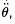as it should, although the coordinate is dimensionless.

Problem 5: Simple pendulum Choose θ as the generalized coordinate for a simple pendulum.
What is an appropriate generalized momentum, so that its time derivative is equal to the force? What is the engineering dimension of the generalized momentum. Draw phase space trajectories for the pendulum: periodic motion corresponds to closed trajectories.
What is the dimension of the area enclosed by such a trajectory?
What is the physical interpretation of this area?

Many particles
Following the motion of N particles requires keeping track of N vectors, x, x2 , · · · , xN . The conﬁguration space has 3N dimensions; the phase space has 6N dimensions. We say that there are 3N degrees of freedom. Phase space volume has engineering dimension of (energy × time)3N .
The equations of motion are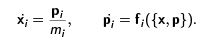If these are subject to some non-holonomic constraints, then there is no reduction in the number of degrees of freedom. If there are M scalar equations expressing holonomic constraints, then the number of degrees of freedom reduces to D = 3N − M . There is a consequent change in the dimension of phase space and the engineering dimension of phase space volume.

Generalized coordinates

If there are M constraints of the form fα (x, x2 , · · · , xN ) = 0 with 1 ≤ α ≤ M , then all the coordinates of the N particles are given in terms of generalized coordinates qi where 1 ≤ i ≤ D = 3N − M .
In other words, one has N vector-valued functions x= xj (q1 , q2 , · · · qD , t ). If the generalized coordinates are to provide a complete description of the dynamics then knowledge of all the qk should be equivalent to specifying all the x. A counting of the number of scalar equations shows that this is possible.
Clearly, the velocities are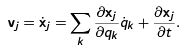As a result one has the important identity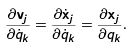Generalized forces

The equations of motion are equivalent to the principle that if one makes an instantaneous virtual displacement of a mechanical system, then the work done by the forces goes into a change of the total kinetic energy. In other words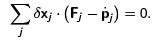Now one can use the generalized coordinates to rewrite the work done by the forces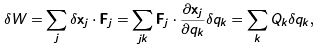where one has deﬁned the generalized forces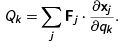The change in kinetic energy

One can write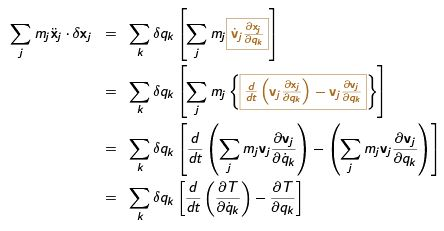Equation of motion

Since the virtual displacements of the generalized coordinates are all independent, one can set each coeﬃcient independently to zero.
Then we have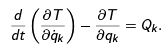If the particles move in a ﬁeld of conservative forces then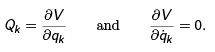Then the equations of motion can be written in terms of the Lagrangian function L = T − V ,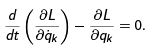Particle in an electromagnetic ﬁeld

The Lorentz force on a particle in an electromagnetic ﬁeld is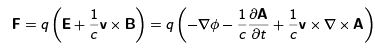where q = charge, c = speed of light, v the velocity, E and B the electric and magnetic ﬁelds, and φ and A the scalar and vector potentials.
The Lagrangian formalism continues to be useful if one can write down a velocity dependent potential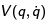such that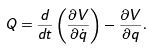Now using the identity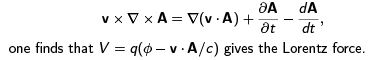Dissipation

The problem of dissipative forces lies a little away from the developments made till now. However, models of frictional forces show that they are proportional to the velocity. Hence, for the dissipative forces on a body one may write the relation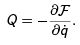This introduces the Rayleigh term,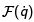, which is usually chosen to be quadratic in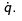The equations of motion are then written as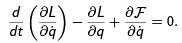In order to describe the motion of a body in a dissipative environment both the Lagrangian L and the Rayleigh term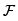need to be speciﬁed.

159 docs

,

,

,

,

,

,

,

,

,

,

,

,

,

,

,

,

,

,

,

,

,

,

,

,

,

,

,

;